## Mertens Conjecture

Given Mertens Function defined by(1)

whereis the Möbius Function, Mertens (1897) conjecture states that(2)

for. The conjecture has important implications, since the truth of any equality of the form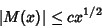(3)

for any fixed(the form of Mertens conjecture with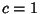) would imply the Riemann Hypothesis. In 1885, Stieltjes claimed that he had a proof that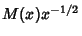always stayed between two fixed bounds. However, it seems likely that Stieltjes was mistaken.

Mertens conjecture was proved false by Odlyzko and te Riele (1985). Their proof is indirect and does not produce a specific counterexample, but it does show that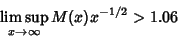(4)(5)

Odlyzko and te Riele (1985) believe that there are no counterexamples to Mertens conjecture for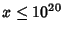, or even. Pintz (1987) subsequently showed that at least one counterexample to the conjecture occurs for, using a weighted integral average ofand a discrete sum involving nontrivial zeros of the Riemann Zeta Function.

It is still not known if(6)

although it seems very probable (Odlyzko and te Riele 1985).

References

Anderson, R. J. On the Mertens Conjecture for Cusp Forms.'' Mathematika 26, 236-249, 1979.

Anderson, R. J. Corrigendum: On the Mertens Conjecture for Cusp Forms.''' Mathematika 27, 261, 1980.

Devlin, K. The Mertens Conjecture.'' Irish Math. Soc. Bull. 17, 29-43, 1986.

Grupp, F. On the Mertens Conjecture for Cusp Forms.'' Mathematika 29, 213-226, 1982.

Jurkat, W. and Peyerimhoff, A. A Constructive Approach to Kronecker Approximation and Its Application to the Mertens Conjecture.'' J. reine angew. Math. 286/287, 322-340, 1976.

Mertens, F. Über eine zahlentheoretische Funktion.'' Sitzungsber. Akad. Wiss. Wien IIa 106, 761-830, 1897.

Odlyzko, A. M. and te Riele, H. J. J. Disproof of the Mertens Conjecture.'' J. reine angew. Math. 357, 138-160, 1985.

Pintz, J. An Effective Disproof of the Mertens Conjecture.'' Astérique 147-148, 325-333 and 346, 1987.

te Riele, H. J. J. `Some Historical and Other Notes About the Mertens Conjecture and Its Recent Disproof.'' Nieuw Arch. Wisk. 3, 237-243, 1985.